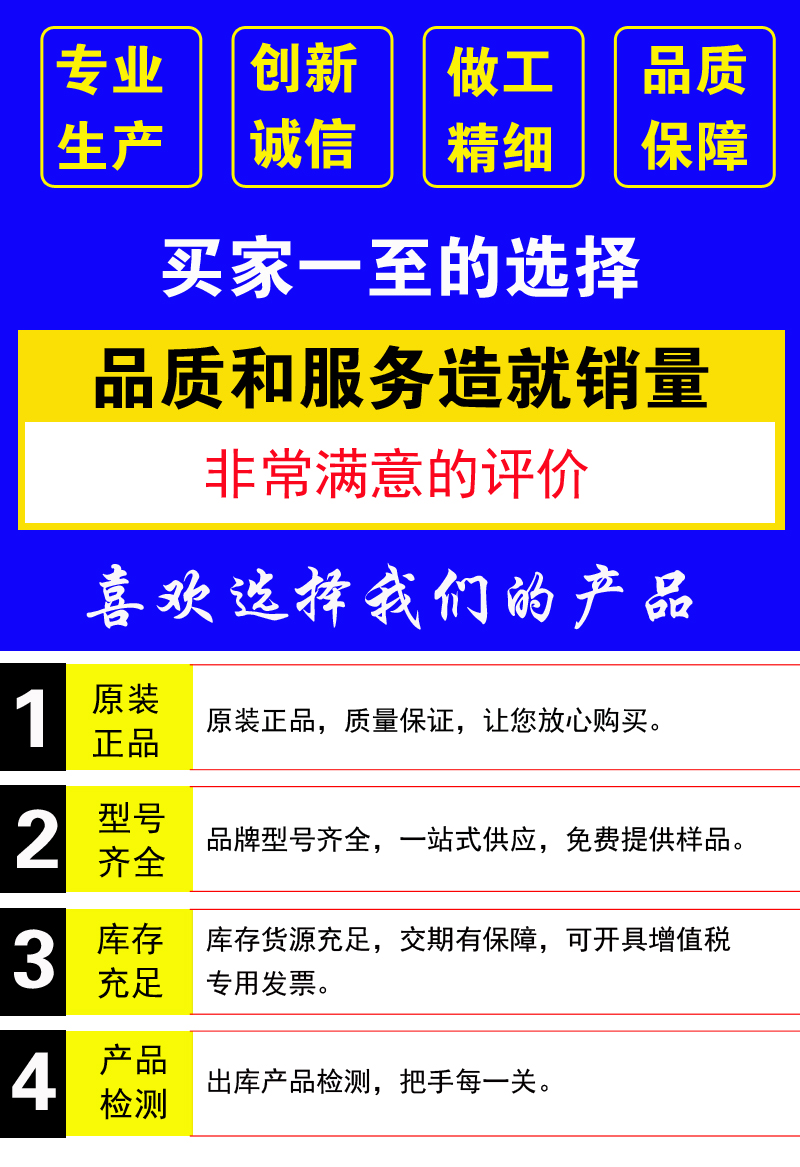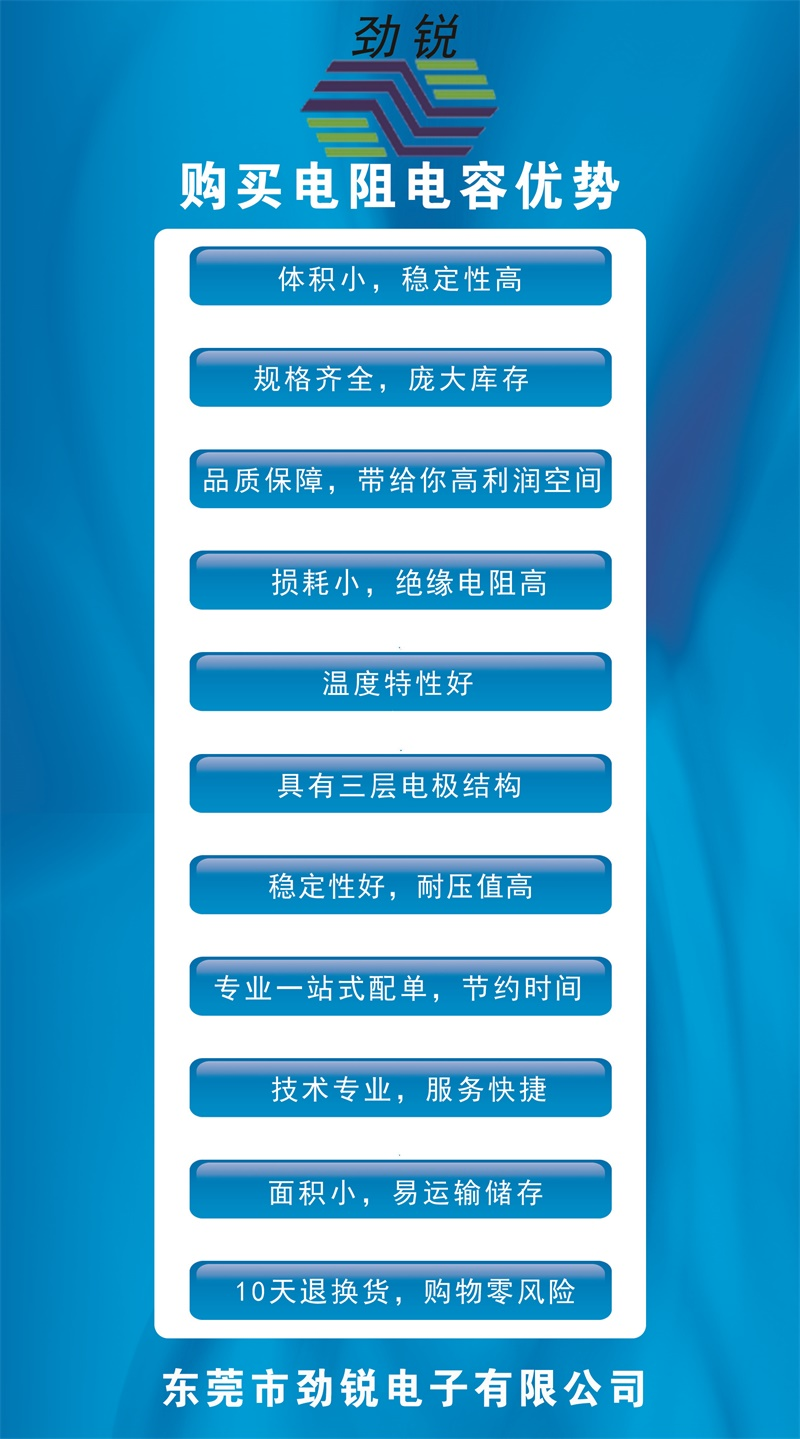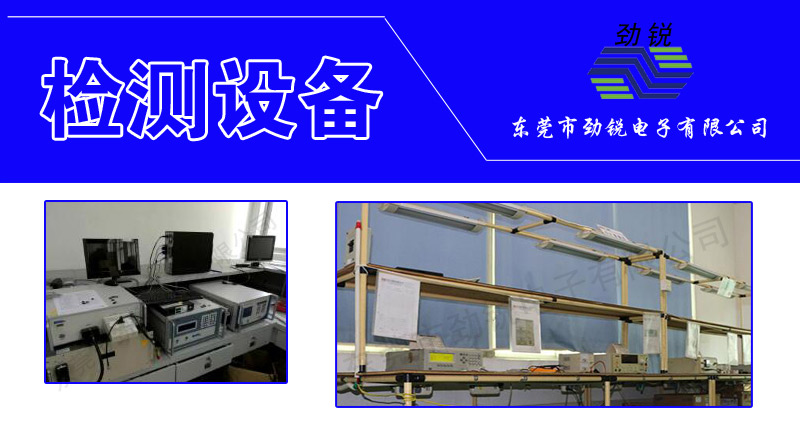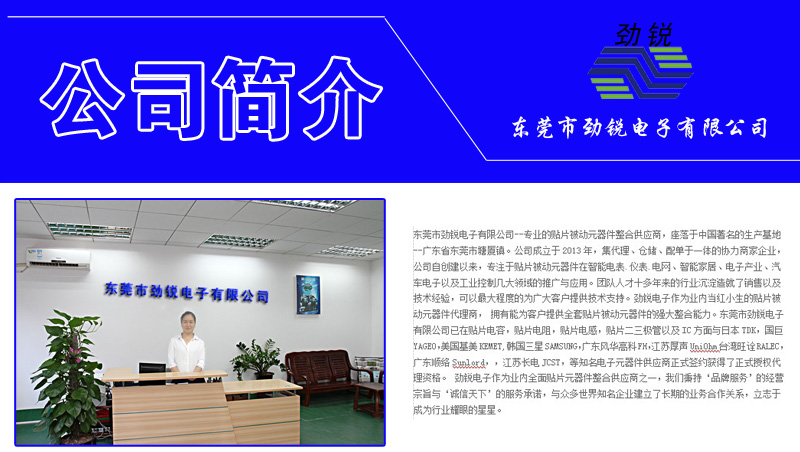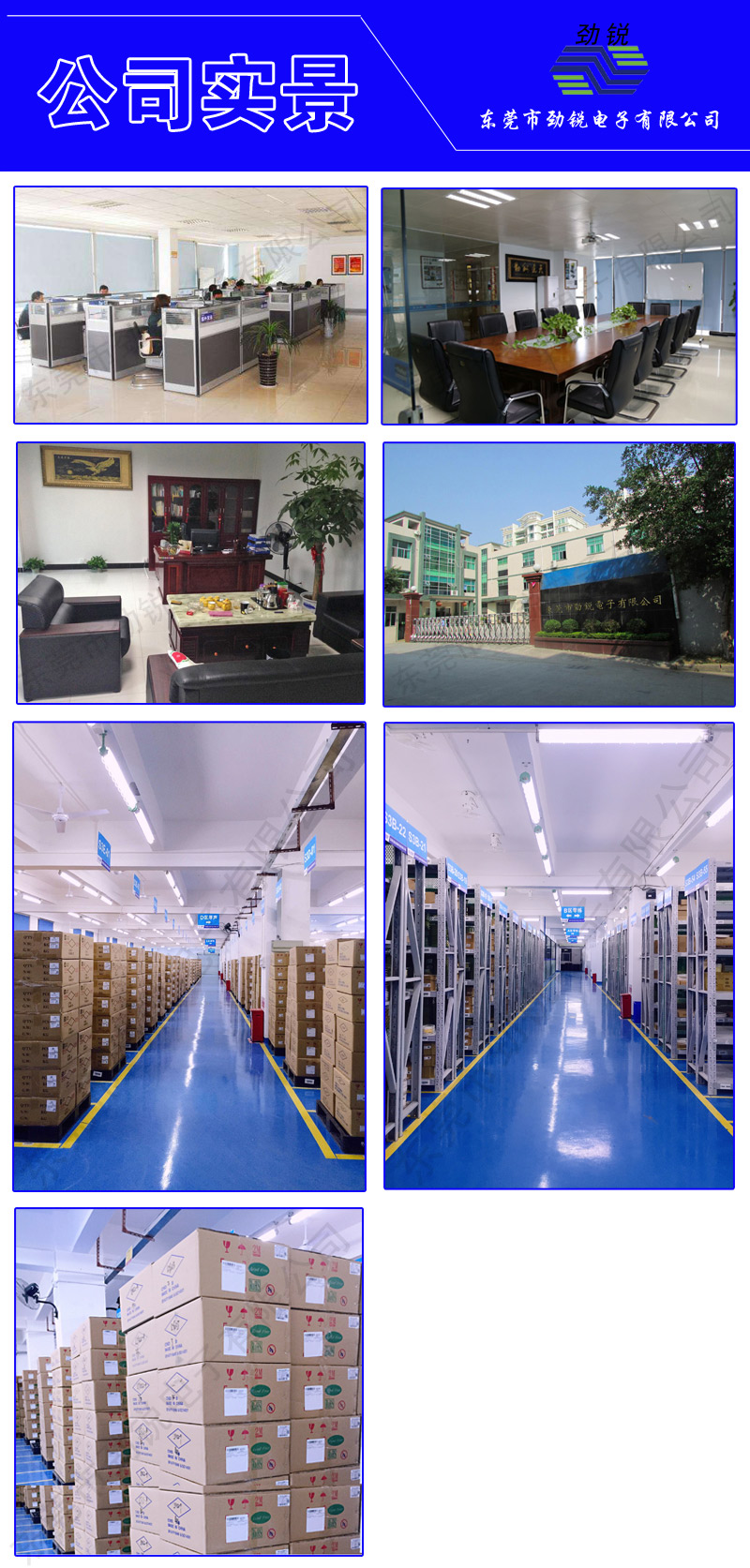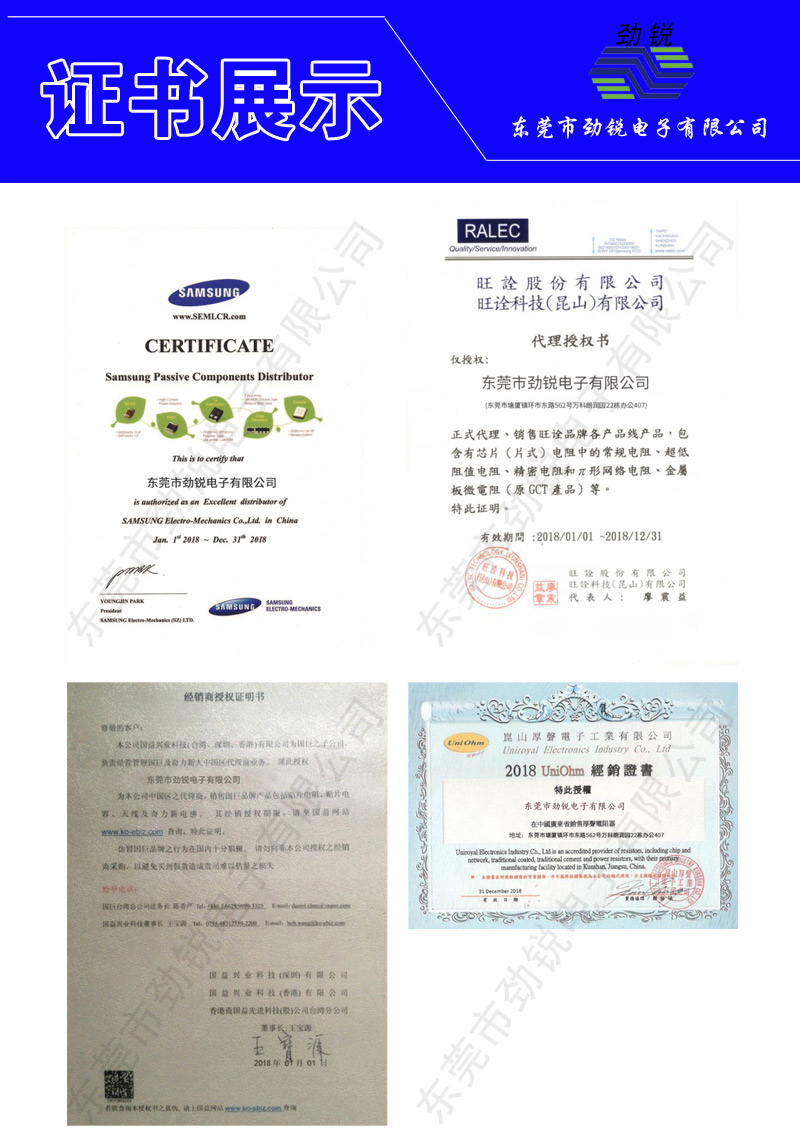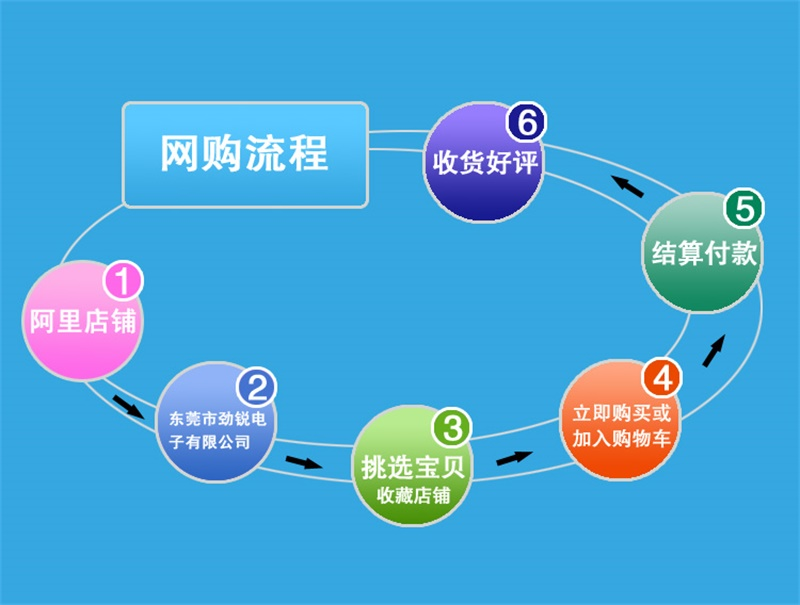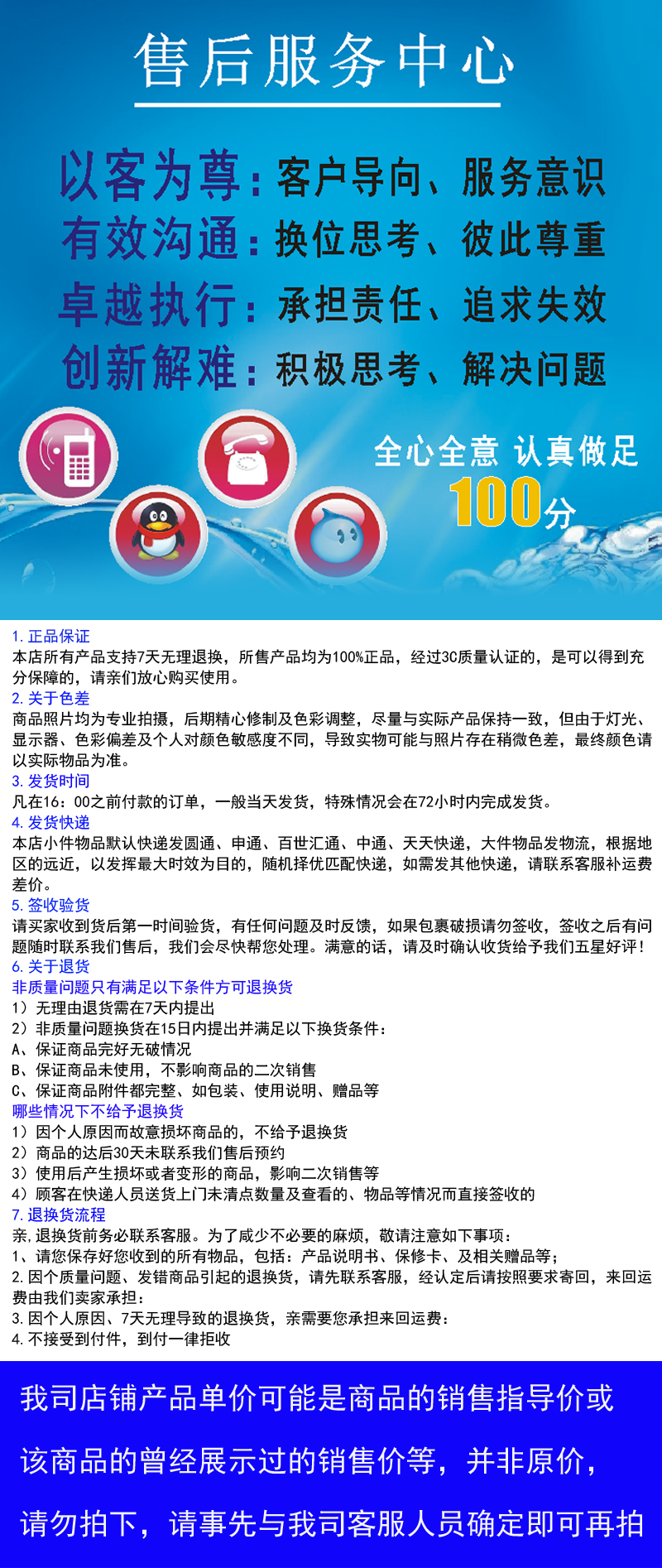Product Categories
4产品展示# 三星CL05B221KBNC

## 详情说明CL=积层陶瓷电容 03=0201(0603) 21=0805(2012) 42=1808(4520)

05=0402(1005) 31=1206(3216) 43=1812(4532) 10=0603(1608) 32=1210(3225) 55=2220(5750) 14=0504(1410)

01=0306(0816) 12=0508(1220)

I类:C=C0G S=S2H L=S2L  P=P2H T=T2H  R=R2H U=U2H

II类:A=X5R  F=Y5V  B=X7R  X=X6S  Y=X7S

P=散装箱 D=纸版箱料带,13英寸料盘(10000ea)  F=压花纸版箱,13英寸料盘 C=纸版箱料带,7英寸料盘  L=纸版箱料带,13英寸料盘(15,000ea) S=压花纸版箱,10英寸料盘。